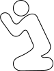CodePlea
Random thoughts on programming
21 Nov 2009

# Optimal Bit Packing

I recently ran into a problem where several values had to be stored in an very minimal amount of space. As an example, we will use four values. Let's call these values,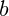,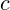, and. Each value has a well defined range, such as: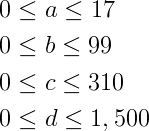Suppose now, that we want to encode all four values into one number. Furthermore, we want to minimize the bandwidth or space this value requires.

Most C programmers know (or at least should know) about bit fields. Bit fields allow a C programmer to set the number of bits required for each integer. For example:

union Pack
{
unsigned int code;
struct
{
unsigned int a : 5;
unsigned int b : 7;
unsigned int c : 9;
unsigned int d : 11;
} packed;
};

Using this method, we can encode all four values into 32 bits. This will greatly reduce the size over the naive method of using a plain int for each value.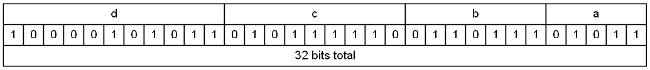The problem here is that each value is actually taking up more space than is absolutely required. For example, we know from our requirements thatwill always be less than 18. Yet, using bit fields we must assign at least 5 bits, which means we could store a value as high as 31. This is wasted space.

Why would anyone worry about wasting a fraction of a bit of memory? Bit packing is only useful when bandwidth or space is extremely limited. For example: applications where a user must key in a value (hopefully encoded in a high base, using letters and numbers). Remember how some old Nintendo games "saved" by having the user write down a string? With bit packing a few characters can be knocked off that string. Similar codes are still used for order processing, check sums, ect. Bit packing can also be useful for encoding information in bar codes.

Optimally packing bits involves only a slight change in the formula. The mathematical formula for our bit field looks like this: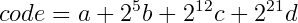It's not hard to see that there is actually no reason to stay on power of 2 boundaries. To maximally pack the values we will simply rewrite the formula using our data's actual boundaries, not arbitrary power of two numbers.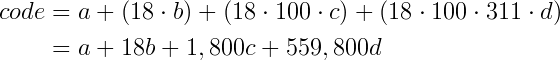Packing and unpacking is still fairly straightforward in C.

unsigned int pack(unsigned int a, unsigned int b,
unsigned int c, unsigned int d)
{
unsigned int code = d;
code *= 311;
code += c;
code *= 100;
code += b;
code *= 18;
code += a;
return code;
}

void unpack(unsigned int code,
unsigned int& a, unsigned int& b,
unsigned int& c, unsigned int& d)
{
a = code % 18;
code /= 18;
b = code % 100;
code /= 100;
c = code % 311;
code /= 311;
d = code;
}

There you have it. What took 32 bits before can now fit in just 30 bits. That's a savings of over 6%. Wow.

Like I mentioned above, that savings can quickly become substantial if there are many values, or if space is at an extreme premium.

Like this post? Consider following me on Twitter or following me on Github. Don't forget to subscribe to my feed.